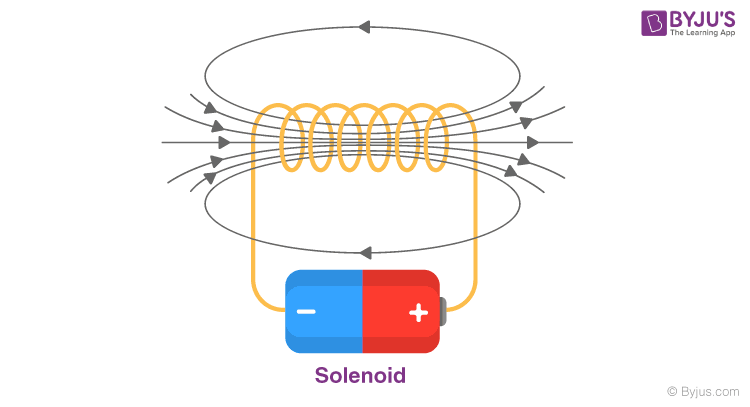# Explain the working of a solenoid

A solenoid is a common term for a wire coil used as an electromagnet. It also applies to any system that uses a solenoid to transform electric energy into mechanical energy. The system generates a magnetic field from electric current and produces linear motion using the magnetic field.

## Working of solenoid

• A solenoid is a coil of wire tied across a piston, mostly made of iron, in the form of a corkscrew.
• Like with all electromagnets, when an electric current passes via the wire a magnetic field is generated.
• The advantage of electromagnets over permanent magnetic material is that they have been turned on and off by adding or removing the electrical field, making them effective as controls and switches, enabling them to be automated.
• The magnetic field of an activated solenoid has both positive and negative poles that attract or repel sensitive material to the magnets.
• The electrical force causes the piston to shift either forward or backward inside a solenoid that’s how a solenoid coil creates motion.

Consider a solenoid of length is such that it is large as compared to its radius. Here the wire is wrapped with a very small gap between any two turns in the shape of the helix. The wires are also enamelled, rendering them insulated from one another. As a consequence, one can take every turn as a closed circular loop. Thus created magnetic field is equal to that formed by a circular loop and the total magnetic field created by the solenoid can be specified as the sum of vector force generated by every such turn. The lines of magnetic fields generated inside a finite solenoid are shown in the figure below.From the above figure, we can see that the magnetic field within the solenoid is constant and runs along the solenoid axis. The field at the outside immediately to the solenoid at every point is very low and the field lines can’t be seen at the close surrounding. It’s necessary to note that at every place the field within is parallel to its axis.

From the Law of the Ampere, the magnetic force created by a solenoid can be described as,

$$F = \mu _{0}nI$$

Where

n = number of turns of the wire per unit length

I = current flowing through the wire

The direction is given using the right-hand thumb rule.(7)(1)